# Team:Tokyo-NoKoGen/modeling

Team:Tokyo-NoKoGen - 2013.igem.org

Modeling

Background

We constructed oscillation circuit using RNA. We think that it is easier to design the system than protein oscillation, and protein oscillator is difficult to control because there are many process such like translation and activation of related proteins. So, it can be used as a very useful tool which has a lot of applications. In our assumption, RNA oscillation using hammerhead ribozyme(HHR) is suitable for our system. But does this system really generate the oscillation? Or, if so, what is the ideal conditions of generation of the oscillation and how much faster than our constructed system is than protein oscillator? To investigate this, we created simple model using Simbiology.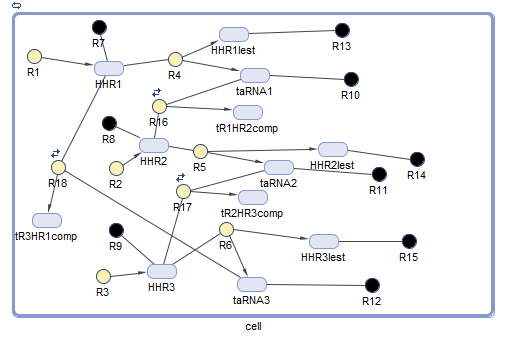Fig1. The scheme of RNA oscillation using Simbiology.

Simbiology is Math Works softare, MATLAB’s extension. This software allows us to simulate biological phenomenon of the species, reactions, and compartments that make up the system (in our system, component of RNA oscillation, such like, HHRs, and taRNAs). For this model, we simulated that how condition this system works (Fig 1).

Method

Before simulation of our construction of RNA oscillator, we estimated the values below: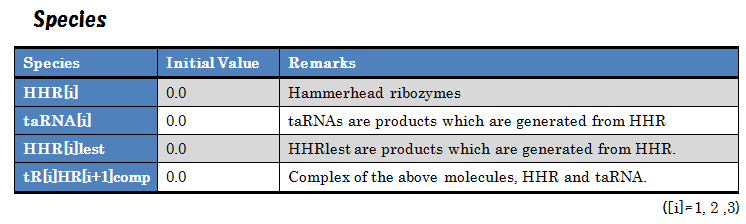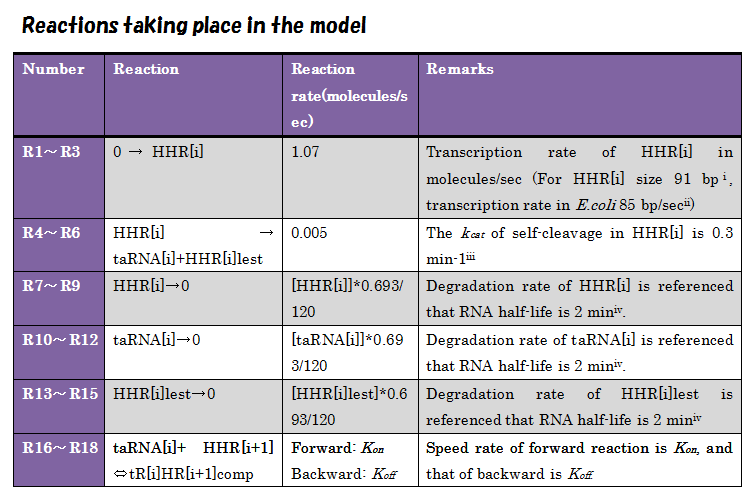We used these values to simulate our RNA oscillation.

Result and Discussion

We used the Simbiology for the simulation, and the result(Kα=0.005, Kon = 2.0, Koff = 1.0) is shown as the graph below.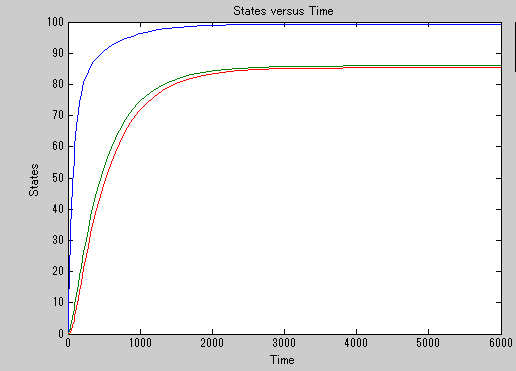Fig2. Time scale of relative amount of HHR1(blue line), taRNA1(green line) and HHR1 and taRNA1 complex(red line).

Results from simulation show that the amount of HHR-taRNA complex, HHR and taRNA reached steady state(X axis means Time(sec), and Y axis means molecules of each species). So, the result shows that this system doesn't generate oscillation. But we substituted false values into Kon and Koff. So, to confirm whether oscillation doesn't occur any time, we formularized differential equations as follows: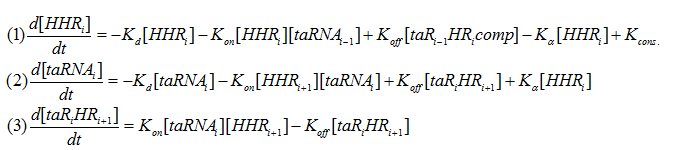where the Kd is the rate of RNA degradation, Kα is rate of self-cleavage frequency, the constant Kcons is the rate of production of new RNA, and [・] is the concentration of "・".(taR[i]HR[i+1] means taRNAi and HRRi+1 complex(i=1,2,3))

To generate oscillation, we focused on the steady state. If oscillation occurs, we’ll get recurrence formula from above differential equations and it shows bi-steady state exists, which is feature of generating oscillation.

In steady state, the changes of each species are 0. Therefore, the following equations are satisfied.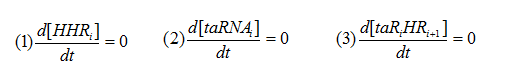And, we get these values immediately as follows,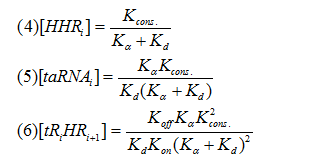From the results (4)～(6) shows that concentration of all of the associated species go to the constant value in steady state, which means that there isn't bi-steady state and that this system doesn't generate the oscillation. When we began this project, we assumed that taRNA inhibits the self-cleavage of HHR and causes a decrease in the amount of HHR. So the oscillation should have occurred. But even when the taRNA inhibits HHR’s activity, HHR itself is continuously expressed and new HHRs come one after another , which leads the concentration of HHR and taRNA to the constant value which depend on Kon, Koffs. Kcons,Kαand Kd. To generate oscillation in our system, we should have introduced the factor which stops transcription directly, such like protein repressor and attenuater.

Reference

i Bnenedikt Klauser and Jorg S. Hartig, (2013), An engineered small RNA-mediated genetic switch based on a ribozyme expression platform, Nucleic Acid Res. ,41(10), 5542-5552
ii Bremer.H., Dennis.P.P(1996) Modulation of chemical composition and other parameters of the cell by growth rate.
iii Gerardo.F., James M. Smith, Robert Cedergren(1998), Schistosome Satellite DNA Encodes Active Hammerhead Ribozymes. Mol Cell Biol. 18(7), 3880-3888
iv Bruce Alberts, et.al, MOLECULAR BIOLOGY OF THE CELL 4th edition (New York, Newton Press, 2004 )